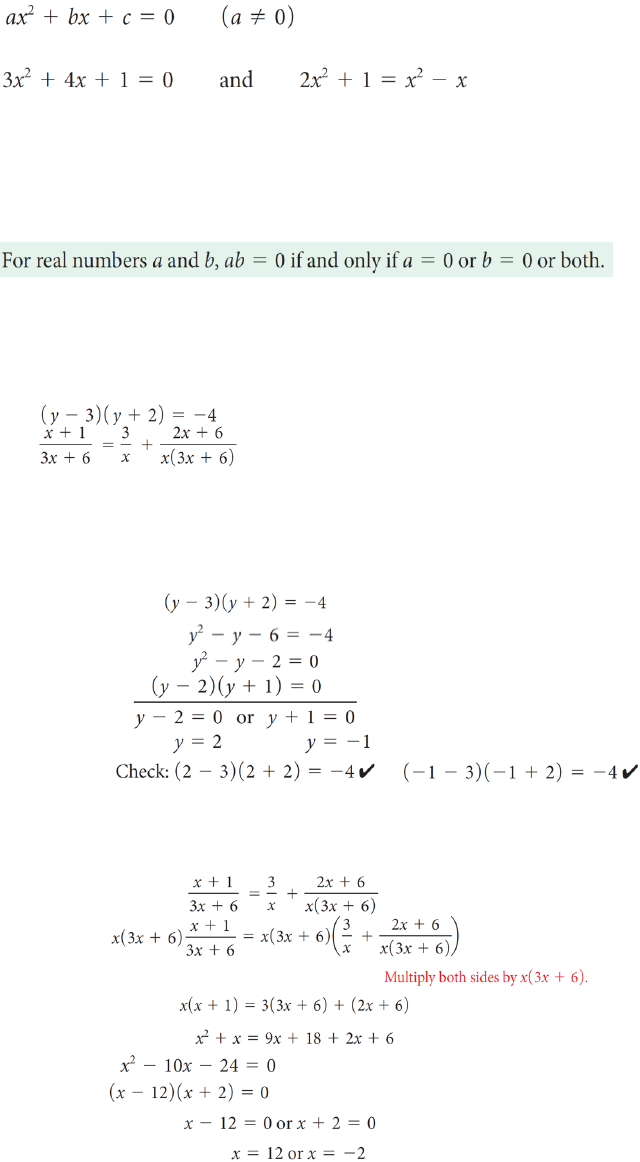# ITM 107 Lecture Notes - Lecture 9: Quadratic Formula, Xterm, Social Security Trust Fund

217 views3 pagesKATIE CHOI
11/5/18
LECTURE 7 QUADRATIC AND OTHER SPECIAL FUNCTIONS
Factoring Methods
A quadratic equation in one variable is an equation that can be written in the general
form
where a, b, and c represent constants. For example, the equations
When we solve quadratic equations, we will be interested only in real number solutions
and will consider two methods of solution: factoring and the quadratic formula.
Solution by factoring is based on the following property of the real numbers.
Zero Product Property
Hence, to solve by factoring, we must first write the equation with zero on one side.
Example Solving by Factoring
Solve:
a)
b)
Solution:
a) Note that the left side of the equation is factored, but the right member is
not 0. Therefore, we must multiply the factors before we can rewrite the
equation in general form.
b) The LCD of all fractions is x (3x + 6). Multiplying both sides of the equation by
this LCD gives a quadratic equation that is equivalent to the original equation
for x ≠ 0 and x 2. (The original equation is undefined for these values.)
Unlock document

This preview shows page 1 of the document.
Unlock all 3 pages and 3 million more documents.

# Get access

\$10 USD/m
Billed \$120 USD annually
Homework Help
Class Notes
Textbook Notes